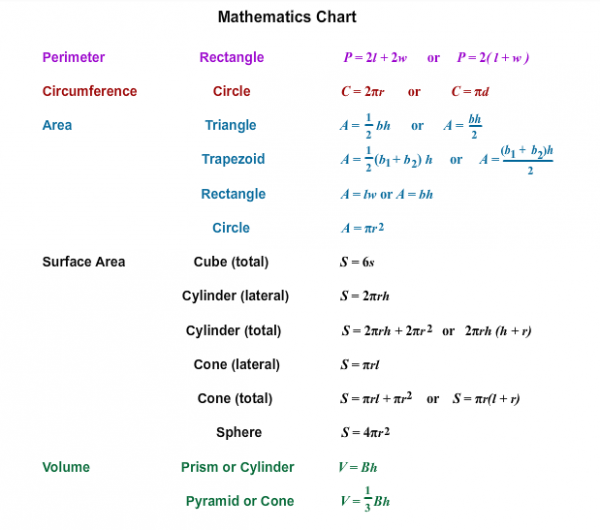# Let's Get Started

You will use the laws of exponents to solve for variables and solve problems.

TEKS Standards and Student Expectations

A(11) Number and algebraic methods. The student applies the mathematical process standards and algebraic methods to rewrite algebraic expressions into equivalent forms. The student is expected to:

A(11)(B) simplify numeric and algebraic expressions using the laws of exponents, including integral and rational exponents

A(12) Number and algebraic methods. The student applies the mathematical process standards and algebraic methods to write, solve, analyze, and evaluate equations, relations, and functions. The student is expected to:

A(12)(E) solve mathematic and scientific formulas, and other literal equations, for a specified variable

Resource Objective(s)

Given problem situations involving exponents, the student will use the laws of exponents to solve the problems.

Essential Questions

How can you solve for a specific variable in a given formula?

What happens to the exponents when you multiply/divide powers with the same base?

Vocabulary

# Laws of Exponents Review

Video

View the video below to review the laws of exponents and how they can be used to solve problems.

# Solving for a Specific Variable Within a Formula

We're going to explore various formulas on a mathematics reference chart to learn how to solve for a specific variable within a formula. Let's take a look at an example of some common formulas used on standardized tests.Find the Formula

Use this mathematics reference chart to find the following formulas. Then match the formulas with the correct shape.

Video

Before using the laws of exponents to solve problems involving formulas, you may have to solve the formula for a specific variable. View the video below to learn more about solving formulas for a specific variable. After viewing the video, complete the following problems. Click on the problem to see the solution.

Source
How to Solve a Formula Equation, tabletclass, YouTube

# Using the Laws of Exponents to Solve Problems

Now you will use formulas and the laws of exponents to solve problems.

Directions: Read each problem and determine the answer to each using the mathematics chart in the previous section and the law of exponents. Click on the plus sign to check the solution.

1. The area of a rectangle measures $36x5y7$. The length of the rectangle measures $4x2y3$. What is the width of the rectangle?

2. The height of a trapezoid is $4ab3$. The bases of the trapezoid are 2ab and 5ab respectively. What is the area of the trapezoid?